Latest SSC jobs   »   Arithmetic Study Notes :   »   Lines and Angles

# Lines and Angles – Definition, Types, Properties and its PDF

## Lines and Angles

Definition of Lines and Angles: Lines and Angles are the basic terms of Geometry. A line is defined as a figure of closely spaced dots that extends indefinitely in two directions. The only one dimension of Line is its length. There are various lines such as, perpendicular lines, transversal lines, intersecting lines, etc. A horizontal mark on a piece of paper is an example of line. An angle is defined as a figure created by two rays that emerge from a common point. All geometry shapes are made up of lines and angles. Lets have a look at the types of lines and angles in this post.

## Lines

A line can be defined as a straight figure which doesn’t have any endpoint and extends infinitely in opposite directions. A line can be horizontal or vertical.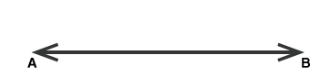## Angles

An angle is formed when line segments meet at a single point i.e. vertex. Angle is measured in degrees (°) or radians and is represented by the symbol ‘∠’.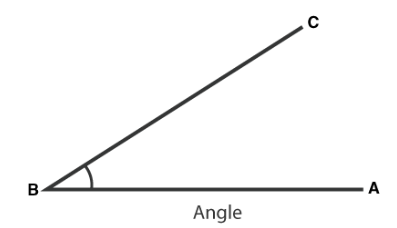## Types of Lines and Angles

In geometry, there are various types of lines and angles based on measurements. The types of Lines and Angles are as follows:

Lines and Angles – Definition, Types, Properties and its PDF In Hindi

### Line Segment

A part of the line formed with two end-points is called a Line Segment. It is the shortest distance between two points.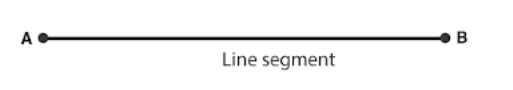### Ray

A ray is a straight line, which has a fixed starting point and moves infinitely in one direction.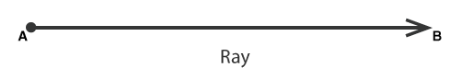### Parallel Lines

When two lines do not never intersects each other or do not meet at any point in a plane are called Parallel Lines.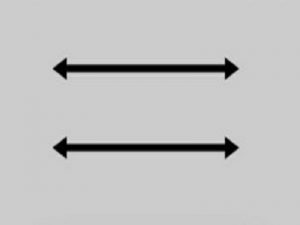### Perpendicular Lines

When two lines meet at a single point and form a right angle, those lines are called perpendicular lines.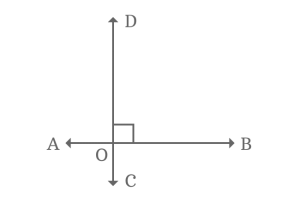### Transversal Line

A line that intersects two or more lines at distinct points is called a transversal line.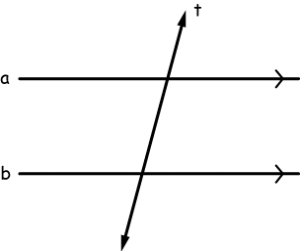## Types of Angles

Angles are classified based on their measurements.

• Acute Angle
• Obtuse Angle
• Right Angle
• Straight Angle

### Acute Angle (<90°)

An angle that measures less than 90 is called an acute angle.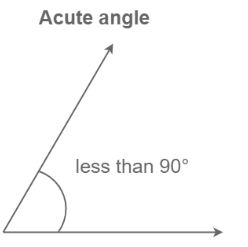### Obtuse Angle (>90°)

An angle that measures between 90° and 180° is called obtuse angle.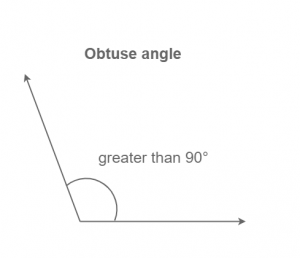### Right Angle (=90°)

An angle that measures equal to 90° is called right angle.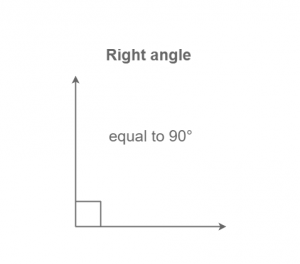### Straight Angle (=180°)

An angle that measures equal to 180 then it is known as a straight angle.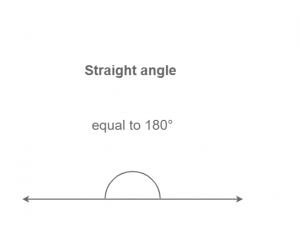## Properties of Lines and Angles

The Properties of Lines and Angles are as follows:

### Properties of Lines

• A line does not have any endpoints.
• The line extends in both the directions infinitely.
• A line has only length, and no thickness.
• Distance between two parallel lines is always the same.
• Intersecting lines cross at only one point.
• Angle between the perpendicular lines is always a right angle.

### Properties of Angles

• An angle is formed when two rays emerge from a common point, called the vertex of the angle and the two rays forming the angle are called its arms or sides.
• When two adjacent angles add up to 180 degrees, they form a linear pair of angles.
 You may also like to read Time, Speed, And Distance BPSC 68th Answer Key Simple Interest Formula Study Notes On Average With Examples

## Lines and Angles Pdf

The Lines and Angles Pdf along with the Lines and Angles Worksheet Pdf will soon be provided here.

### Lines and Angles: FAQs

Q. What is the definition of Line?

Ans. A line can be defined as a straight figure which doesn’t have any endpoint and extends infinitely in opposite directions. A line can be horizontal or vertical.

Q. What is a Ray?

Ans. A ray is a straight line, which has a fixed starting point and moves infinitely in one direction.

Q. What are the types of Angles?

Ans. Types of Angles are:

• Acute Angle
• Obtuse Angle
• Right Angle
• Straight Angle

## FAQs

### Q. What is the definition of Line?

Ans. A line can be defined as a straight figure which doesn’t have any endpoint and extends infinitely in opposite directions. A line can be horizontal or vertical.

### Q. What is a Ray?

Ans. A ray is a straight line, which has a fixed starting point and moves infinitely in one direction.

### Q. What are the types of Angles?

Ans. Types of Angles are:

Acute Angle
Obtuse Angle
Right Angle
Straight Angle

#### Congratulations!General Awareness & Science Capsule PDFIncorrect details? Fill the form again here

General Awareness & Science Capsule PDF

Thank You, Your details have been submitted we will get back to you.

### TOPICS:

•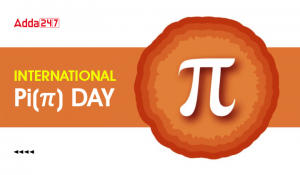International Pi Day 2023, All You Need ...
•Study Notes On Average Formula With Exam...
•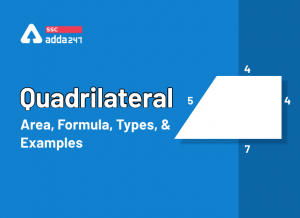Quadrilateral Area, Formula, Types, Prop...
•Profit and Loss Formula, Concept and Stu...
•Mixture and Alligation, Notes, Methods a...
•Speed, Time And Distance Tricks and Ques...